## Continuity Equation in Semiconductor:

The Continuity Equation in Semiconductor states a condition of dynamic equilibrium for the concentration of mobile carriers in any elementary volume of the semiconductor.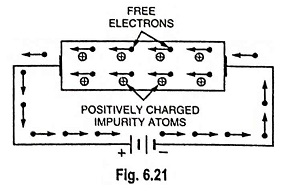In Fig. 6.21 we have seen that on disturbing the equilibrium concentrations of carriers (electrons and holes) in a semiconductor, the concentrations of holes or electrons vary with time approaching the equilibrium value exponentially. In the general case, however, the carrier concentration in the body of a semiconductor is a function of both time and distance. The differential equation governing this functional relationship, called the Continuity Equation in Semiconductor, is based upon the fact that charge can be neither created nor destroyed.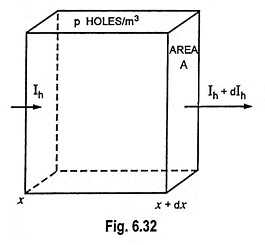Consider an infinitesimal element of volume of area A and length dx, as shown in Fig. 6.32, within which the average hole concentration is p. If τh is the mean lifetime of holes, then p/τh equals the holes per second lost by recombination per unit volume. If e is the electronic charge, then, because of recombination, the number of coulombs per second decreases within the volume and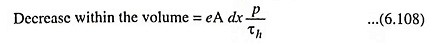If g is the thermal rate of generation of electron-hole pairs per unit volume, the number of coulombs per second increases within the volume and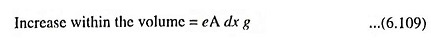In general, the current varies with distance within the semiconductor. If the current entering the volume at x is Ih and leaving at x + dx is Ih + dlh, as shown in Fig. 6.32, the number of coulombs per second decreases within the volume and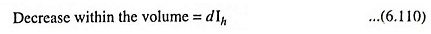Because of the three effects enumerated above, the hole concentration must change with time, and the total number of coulombs per second increases within the volume and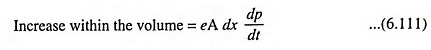Since the charge must be conserved,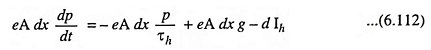The hole current Ih is the sum of drift current and diffusion current so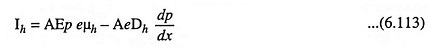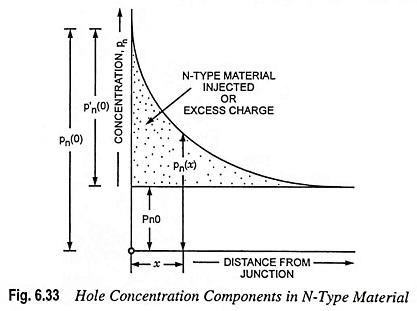If the semiconductor is in thermal equilibrium with its surroundings and is subjected to no applied fields, the hole density will attain a constant value p0. Under these conditions, Ih = 0 and dp/dt = 0. So from the Eq. (6.112), we have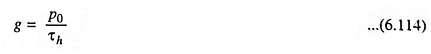The above equation indicates that the rate of thermal generation of holes equals the rate of holes lost due to recombination, under equilibrium conditions.

Combining Eqs. (6.112), (6.113) and (6.114) we have the equation of conservation of charge, called the continuity equation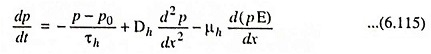The Eq. (6.115) is the continuation equation or equation of conservation of charge for holes stating the condition of dynamic equilibrium for the density of mobile carrier holes. Since p is a function of both t and x, partial derivatives should be used and Eq. (6.115) may be modified as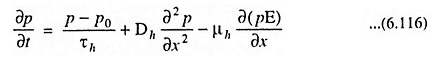Similarly, the Continuity Equation in Semiconductor for electrons states the condition of dynamic equilibrium for the density of mobile carrier electrons and is given by

Scroll to Top